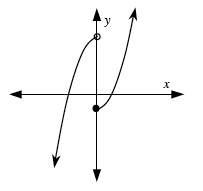### Home > PC > Chapter 4 > Lesson 4.1.3 > Problem4-44

4-44.

Sketch the graph of $f ( x ) = \left\{ \begin{array} { c l } { - x ^ { 2 } + 4 } & { \text { for } x < 0 } \\ { x ^ { 2 } - 1 } & { \text { for } x \geq 0 } \end{array} \right.$. Is $f(x)$ continuous? Explain.

1. Find the height at $0$ for both parts of the piece-wise function.
2. If they are the same, it is continuous.
3. Plot the points found above and continue with the appropriate graph on either side.